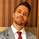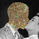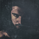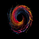# Elastic Volume Weighted Moving Average & Envelope [LazyBear]29935 views
Elastic Volume Weighted Moving Average (eVWMA) is a statistical measure using the volume to define the period of the moving average. The eVWMA can be looked at as an approximation to the average price paid per share. Multiplier is usually the number of shares, but it can be approximated using cumulative sum of volume (Enable it via "Use Cumulative Volume" option) or sum of volume over "n" periods.

I have also added an option to draw eVWMA envelope (eVWMA on HLC ).

http://christian-fries.de/evwma/PDF/evwm...

List of all my indicators:
Open-source script

In true TradingView spirit, the author of this script has published it open-source, so traders can understand and verify it. Cheers to the author! You may use it for free, but reuse of this code in a publication is governed by House Rules. You can favorite it to use it on a chart.

Want to use this script on a chart?
```//
// @author LazyBear
// List of all my indicators: https://www.tradingview.com/v/4IneGo8h/
//
study("Elastic Volume Weighted Moving Average [LazyBear]", shorttitle="EVWMA_LB", overlay=true)
length=input(20)
useCV=input(false, type=bool, title="Use Cumulative Volume")
renderBands=input(false, type=bool, title="Draw Envelope")
nbfs = useCV ? cum(volume) : sum(volume, length)
medianSrc=close
calc_evwma(price, length, nb_floating_shares) =>
data = (nz(data) * (nb_floating_shares - volume)/nb_floating_shares) + (volume*price/nb_floating_shares)
data

m=calc_evwma(medianSrc, length, nbfs)
plot(m, color=maroon, linewidth=2, title="evwma")
plot(renderBands ? calc_evwma(high, length, nbfs): na, color=red  , linewidth=2, title="evwma+")
plot(renderBands ? calc_evwma(low, length, nbfs) : na, color=green, linewidth=2, title="evwma-")```
List of my free indicators: http://bit.ly/1LQaPK8
List of my indicators at Appstore: http://blog.tradingview.com/?p=970This calculation isn't anything new, it is simply the TRUE calculation of the volume weighted moving average. Trading view calculates the VWMA incorrectly as instructed by their own documentation when you search for the vwma() function.

This formula here shows the step-by-step calculation of the VWMA as an incremental/rolling calculation: http://people.ds.cam.ac.uk/fanf2/hermes/doc/antiforgery/stats.pdf, Eq 4-53

U = Un-1 + w / Wn * (x - Un-1)

let Wn = nb_floating_shares (sum of volume (weight) N bars back)
let w = current volume (current weight)
let x = price
let Un-1 = previous MA calculation
let Un = current MA

from line 12 of this script in the calc_evwma function, substituting the above variables:

U = Un-1 * (Wn - w) / Wn + (w * x / Wn)
= Un-1 / Wn * (Wn - w) + w * x / Wn
= Un-1 - Un-1 * w / Wn + w * x / Wn
= Un-1 + w / Wn * (x - Un-1)

This is the exact same formula in the final value in Equation 4-53 above. This is also the same calculation here https://www.tradingsetupsreview.com/volume-weighted-moving-average-vwma/

The Trading View calculation of vwma is wrong, it should be the above. The elastic volume weighted moving average is simply another form of the normal VWMA formula, it is not a new moving average.mortdiggiddy
@mortdiggiddy, so what you're saying is that this script is the true implementation of the VWMA (using cumulative volume or the sum of the volume over a specified length?) and the code above is the correct implementation of the eVWMA?

Sorry to be extra-dense, but would it be possible for you to script-up the correct implementation of the eVWMA (or whatever it is you've commented above)?

And thank you for sharing those articles. I love when people leave gold in the comments.mortdiggiddy
@mortdiggiddy, Indeed, If you plot the cumulative sum version (All timeSeries from within it's origin price) side by side with VWAP, you will have quite much the same result. Tony Finch's paper is a jewel, it defines and proofs why this kind of incremental rolling could have better computation performance in their AutoRegressive algorithm model. (EWMA for math-statistics, 1-pole Infinite Impulse Response for math-frequentists). Can't remember name of paper for the computational proof for this, but google is always your friend. ;)mortdiggiddy
@mortdiggiddy, I’m new here, so please correct me if I’m wrong.

According to the definition of eVWMA presented in the paper, “Wn” here should be a constant, i.e. total number of shares, that doesn’t have to do with sum of Wi (which would be the total volumes of interest). With Wn != sum of Wi, the equations above do not check out. Hence, it’s more like a volume weighted “cumulative” average (or a special case of VWMA, where all historical volumes are considered), plus a decaying effect on previous trades. Does it make sense?